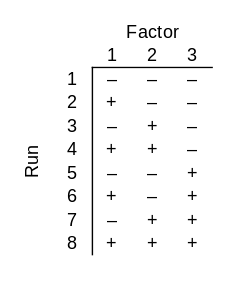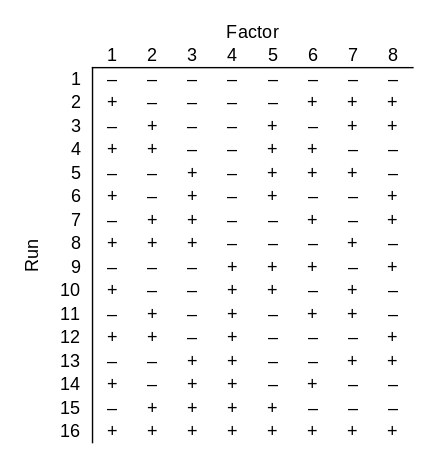# Efficiently testing a black box

Suppose you have a black box that takes three bits as input and produces one bit as output. You could think of the input bits as positions of toggle switches, and the output bit as a light attached to the box that is either on or off.

## Full factorial design

Now suppose that only one combination of 3 bits produces a successful output. There’s one way to set the switches that makes the light turn on. You can find the successful input by brute force if you test all 2³ = 8 possible inputs. In statistical lingo, you are conducting an experiment with a factorial design, i.e. you test all combinations of inputs.In the chart above, each row is an experimental run and each column is a bit. I used – and + rather than 0 and 1 because it is conventional in this topic to use a minus sign to indicate that a factor is not present and a plus sign to indicate that it is present.

## Fractional factorial design

Now suppose your black box takes 4 bits as inputs, but only 3 of them matter. One of the bits does nothing, but you don’t know which bit that is. You could use a factorial design again, testing all 24 = 16 possible inputs. But there’s a more clever approach that requires only 8 guesses. In statistical jargon, this is a fractional factorial design.No matter which three bits the output depends on, all combinations of those three bits will be tested. Said another way, if you delete any one of the four columns, the remaining columns contain all combinations of those bits.

## Replications

Now suppose your black box takes 8 bits. Again only 3 bits matter, but you don’t know which 3. How many runs do you need to do to be certain of finding the successful combination of 3 bits? It’s clear that you need at least 8 runs: if you know that the first three bits are the important ones, for example, you still need 8 runs. And it’s also clear that you could go back to brute force and try all 28 = 256 possible inputs, but the example above raises your hopes that you could get by with less than 256 runs. Could you still get by with 8? That’s too much to hope for, but you could use only 16 runs.Note that since this design works for 8 factors, it also works for fewer factors. If you had 5, 6, or 7 factors, you could use the first 5, 6, or 7 columns of the design above.

This design has some redundancy: every combination of three particular bits is tested twice. This is unfortunate in our setting because we are assuming the black box is deterministic: the right combination of switches will always turn the light on. But what if the right combination of switches probably turns on the light? Then redundancy is a good thing. If there’s an 80% chance that the right combination will work, then there’s a 96% chance that at least one of the two tests of the right combination will work.

Fractional factorial experiment designs are usually used in with the assumption that there are random effects, and so redundancy is a good thing.

You want to test each main effect, i.e. each single bit, and interaction effects, i.e. combinations of bits, such as pairs of bits or triples of bits. But you assume that not all possible interactions are important; otherwise you’d need a full factorial design. You typically hit diminishing return with interactions quickly: pairs of effects are often important, combinations of three effects are less common, and rarely would an experiment consider forth order interactions.

If only main effects and pairs of main effects matter, and you have a moderately large number of factors n, a fractional factorial design can let you use a lot less than 2n runs while giving you as many replications of main and interaction effects as you want.

## Verification

The following Python code verifies that the designs above have the claimed properties.

```    import numpy as np
from itertools import combinations

def verify(matrix, k):
"verify that ever choice of k columns has 2^k unique rows"
nrows, ncols = matrix.shape
for (a, b, c) in combinations(range(ncols), k):
s = set()
for r in range(nrows):
if len(s) != 2**k:
print("problem with columns", a, b, c)
print("number of unique elements: ", len(s))
print("should be", 2**k)
return
print("pass")

m = [
[-1, -1, -1, -1],
[-1, -1, +1, +1],
[-1, +1, -1, +1],
[-1, +1, +1, -1],
[+1, -1, -1, +1],
[+1, -1, +1, -1],
[+1, +1, -1, -1],
[+1, +1, +1, +1]
]

verify(np.matrix(m), 3)

m = [
[-1, -1, -1, -1, -1, -1, -1, -1],
[+1, -1, -1, -1, -1, +1, +1, +1],
[-1, +1, -1, -1, +1, -1, +1, +1],
[+1, +1, -1, -1, +1, +1, -1, -1],
[-1, -1, +1, -1, +1, +1, +1, -1],
[+1, -1, +1, -1, +1, -1, -1, +1],
[-1, +1, +1, -1, -1, +1, -1, +1],
[+1, +1, +1, -1, -1, -1, +1, -1],
[-1, -1, -1, +1, +1, +1, -1, +1],
[+1, -1, -1, +1, +1, -1, +1, -1],
[-1, +1, -1, +1, -1, +1, +1, -1],
[+1, +1, -1, +1, -1, -1, -1, +1],
[-1, -1, +1, +1, -1, -1, +1, +1],
[+1, -1, +1, +1, -1, +1, -1, -1],
[-1, +1, +1, +1, +1, -1, -1, -1],
[+1, +1, +1, +1, +1, +1, +1, +1],
]

verify(np.matrix(m), 3)
```

## One thought on “Efficiently testing a black box”

1. For a use case like the one in the first paragraph with a black box that is deterministic and where you only care about hitting _every_ combination for _all_ sets of t input bits at least once and not about any replication or testing each combination an equal number of times, the term to find literature on how many input vectors you need and how to generate them is “covering array”.

I was looking for this, remembered this blog post, but when I searched for “fractional factorial design” the things I found at first didn’t cover these aspects.